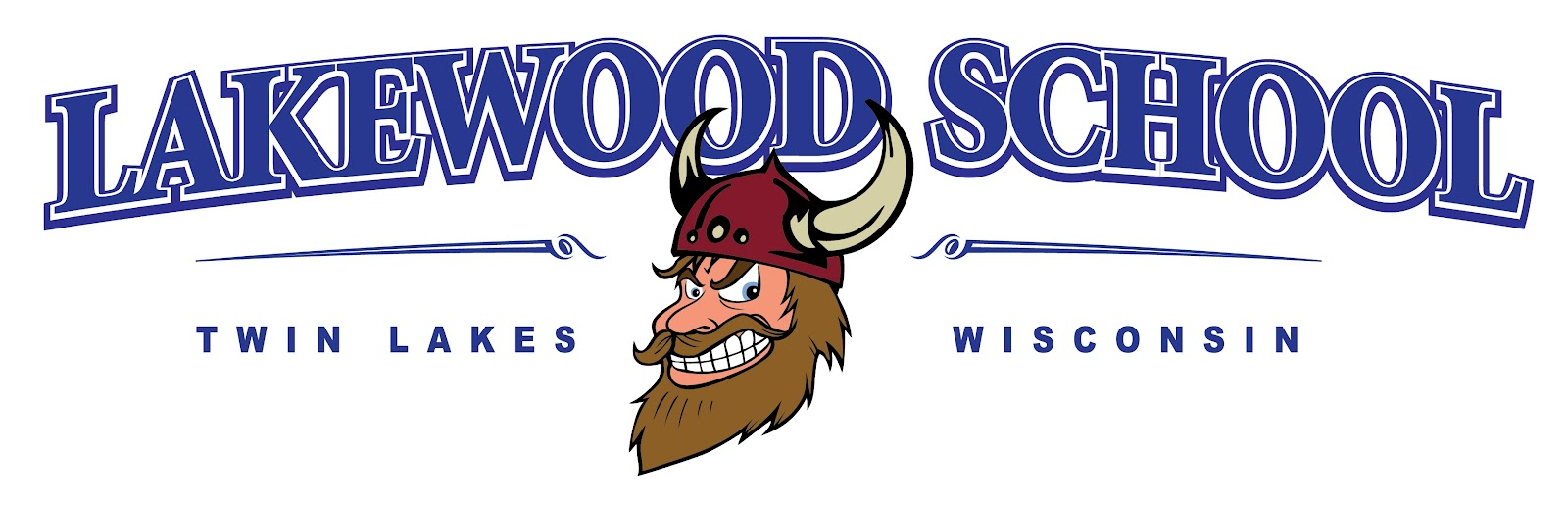First Grade Curriculum Printable ELA English, Language Arts I can hear the difference between long and short vowel sounds. I can listen to sounds and put them together to make a word. I can hear and say the beginning, middle and ending sound in a word. I can hear a word and break it into individual letter sounds. I can read words with common digraphs. Ex. ch, sh I can decode one syllable words. I know final -e and common vowel team conventions for representing long vowel sounds. I can count the syllables in a word using vowels. I can decode two syllable words with short vowels. I can read words ending in -s, -es, -ed, -ing. I can read 1st grade sight words. I can read on-level text fluently. I use context to help me read and self-correct if necessary. I can ask and answer questions about key details in a text. I can retell stories with key details and message. I can describe characters, settings, and major events in a story, using key details. I can explain the difference between fiction and nonfiction text. I can Identify who is telling the story at various points in a text. I can compare and contrast the adventures and experiences of characters in stories. I can Identify the main topic and key details of a text. I can compare two pieces of information in a text. I can use text features to locate information. I can Identify the reasons an author gives to support points in a text. I can compare and contrast two texts of the same topic. I can introduce a topic, supply a reason for my opinion and give my piece closure. I can supply facts about my topic and give my piece closure. I can write about two or more events with details, use sequence words and give my piece closure. MATH Operations and Algebraic Thinking, Numbers and Operations in Base Ten, Measurement and Data, Geometry Use addition and subtraction within 20 to solve word problems involving situations of adding to, taking from, putting together, taking apart, and comparing, with unknowns in all positions Solve word problems that call for addition of three whole numbers whose sum is less than or equal to 20 Apply properties of operations as strategies to add and subtract Understand subtraction as an unknown-addend problem Relate counting to addition and subtraction (e.g., by counting on 2 to add 2) Add and subtract within 20, demonstrating fluency for addition and subtraction within 10 Understand the meaning of the equal sign, and determine if equations involving addition and subtraction are true or false Count to 120, starting at any number less than 120 Read and write numerals and represent a number of objects with a written numeral Understand that the two digits of a two-digit number represent amounts of tens and ones Compare two two-digit numbers based on meanings of the tens and ones digits, recording the results of comparisons with the symbols >, =, and < Add within 100, including adding a two-digit number and a one-digit number, and adding a two-digit number and a multiple of 10 Understand that in adding two-digit numbers, one adds tens and tens, ones and ones; and sometimes it is necessary to compose a ten Given a two-digit number, mentally find 10 more or 10 less than the number, without having to count; explain the reasoning used Subtract multiples of 10 in the range 10-90 from multiples of 10 in the range 10-90 Order three objects by length; compare the lengths of two objects indirectly by using a third object Express the length of an object as a whole number of length units, by laying multiple copies of a shorter object (the length unit) end to end Tell and write time in hours and half-hours using analog and digital clocks Organize, represent, and interpret data with up to three categories Ask and answer questions about the total number of data points, how many in each category, and how many more or less are in one category than in another Distinguish between defining attributes (e.g., triangles are closed and three-sided) versus non-defining attributes (e.g., color, orientation, overall size) Build and draw shapes to possess defining attributes Compose two-dimensional shapes or three-dimensional shapes Partition circles and rectangles into two and four equal shares, describe the shares using the words halves, fourths, and quarters, and use the phrases half of, fourth of, and quarter of Describe the whole as two of, or four of the shares